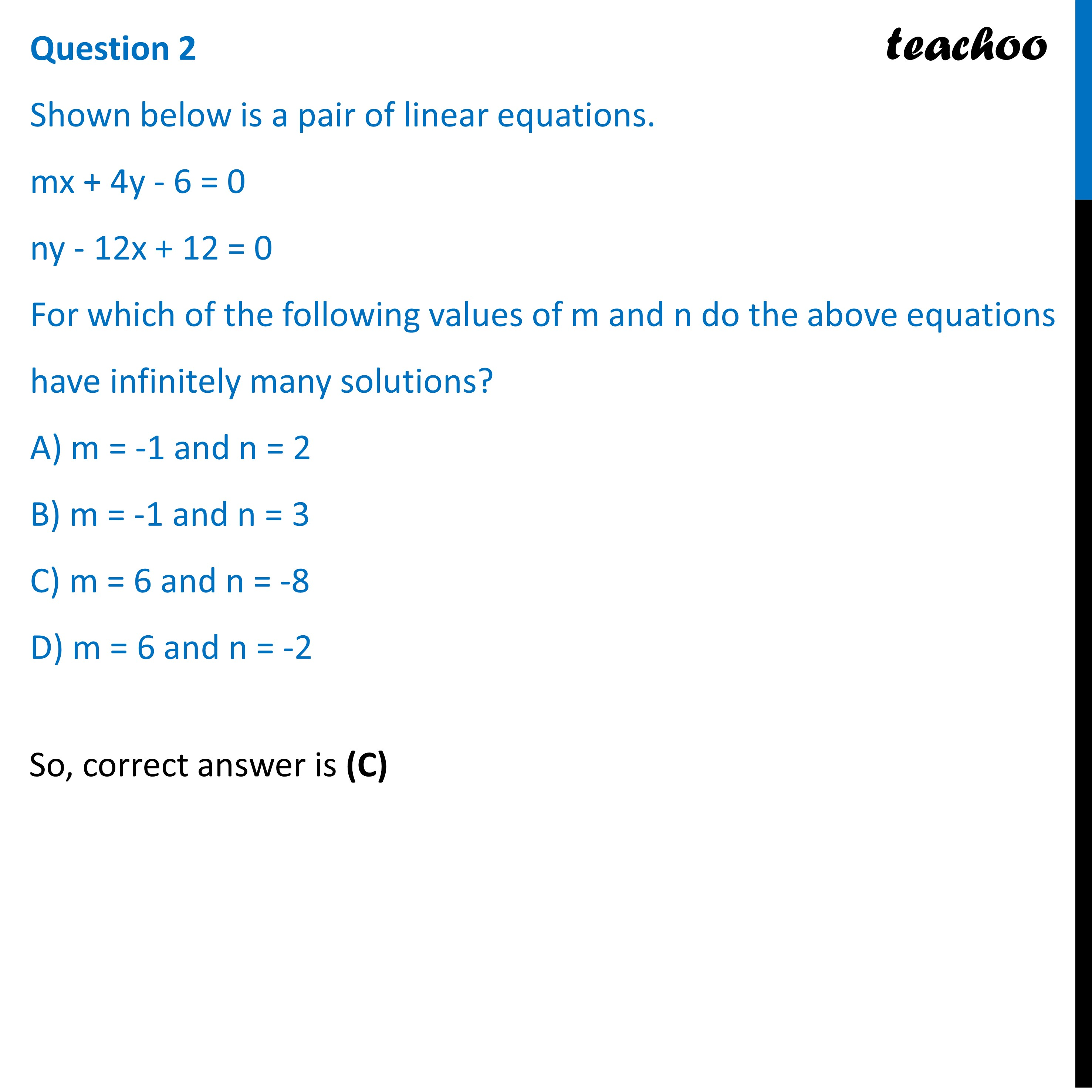Practice Questions CBSE - Maths Class 10 (2023 Boards)

Class 10
Solutions of Sample Papers for Class 10 Boards

## D) m = 6 and n = -2Learn in your speed, with individual attention - Teachoo Maths 1-on-1 Class

### Transcript

Shown below is a pair of linear equations. mx + 4y - 6 = 0 ny - 12x + 12 = 0 For which of the following values of m and n do the above equations have infinitely many solutions? A) m = -1 and n = 2 B) m = -1 and n = 3 C) m = 6 and n = -8 D) m = 6 and n = -2